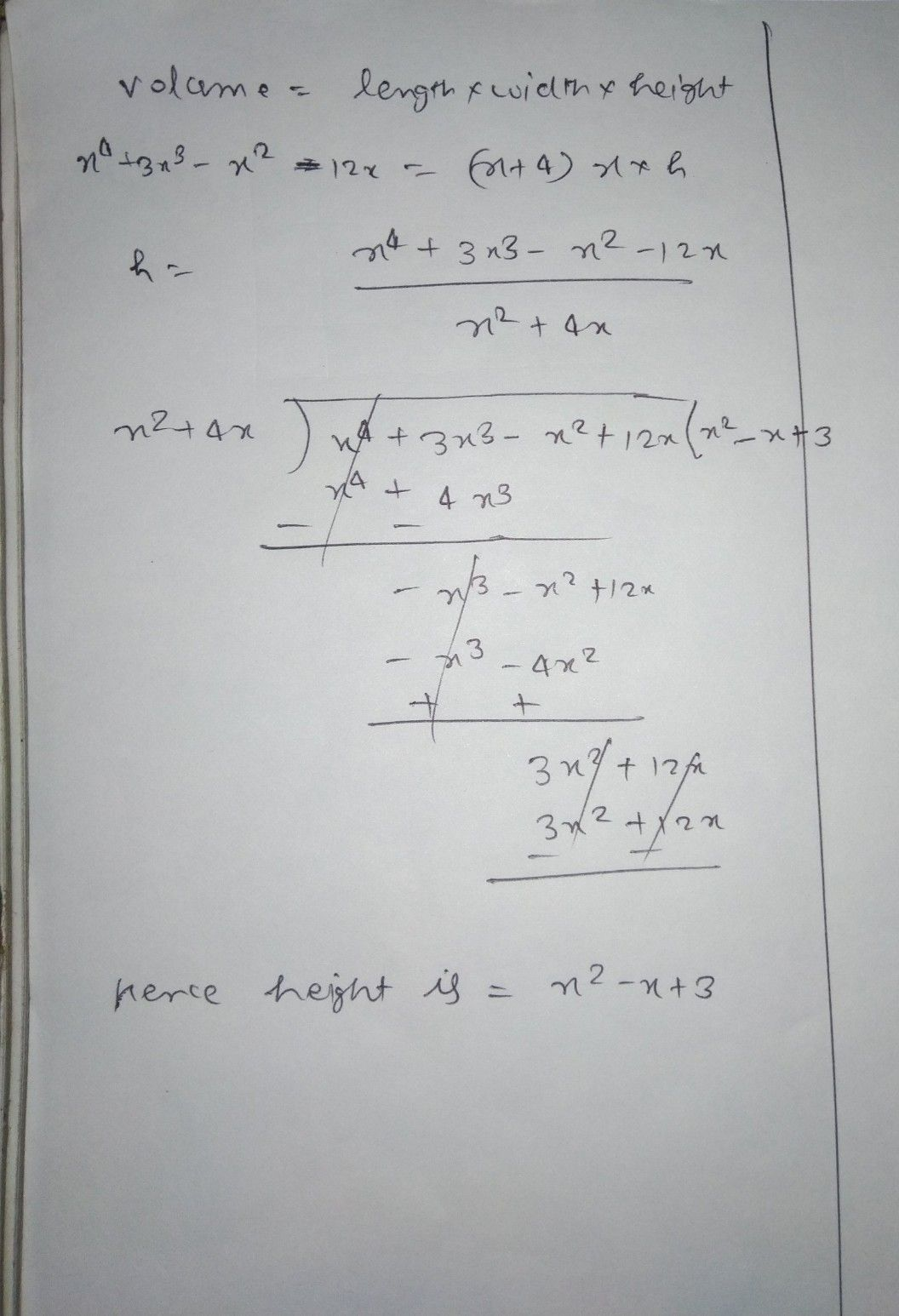Symbol
ProblemRead and analyze the problem carefully. Write your answer on a separate sheet of paper. Show your complete solution. $1.$ The volume of the rectangular solid is given by the polynomial $x^{4}+3x^{3}-x^{2}=12x$ The length $1sx+4$ and the width is x. What is the height of the solid?
1st-6th grade
Other
Search count: 112
SolutionQanda teacher - manish